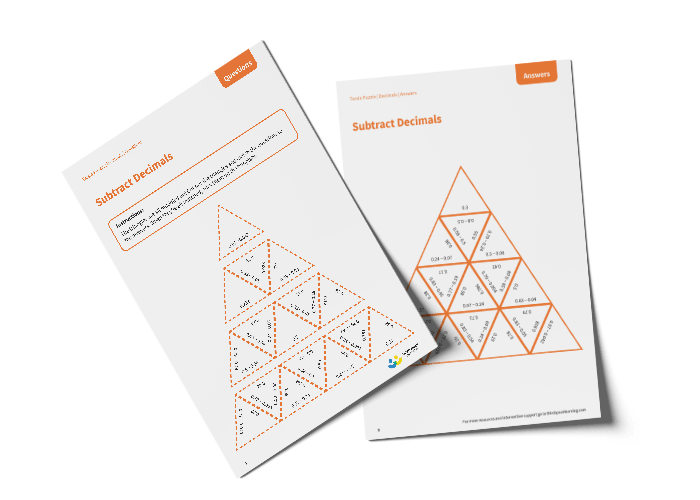# Tarsia Puzzle Subtract Decimals (Year 5)

The triangles in the tarsia puzzle have all been mixed up. In this subtracting decimals tarsia puzzle, pupils will need to match the subtraction question to the correct answer to create one large triangle.

This Year 5 subtract decimals tarsia puzzle worksheet contains:

• One question sheet
• One template sheet

Year 5

Subtraction, Decimals